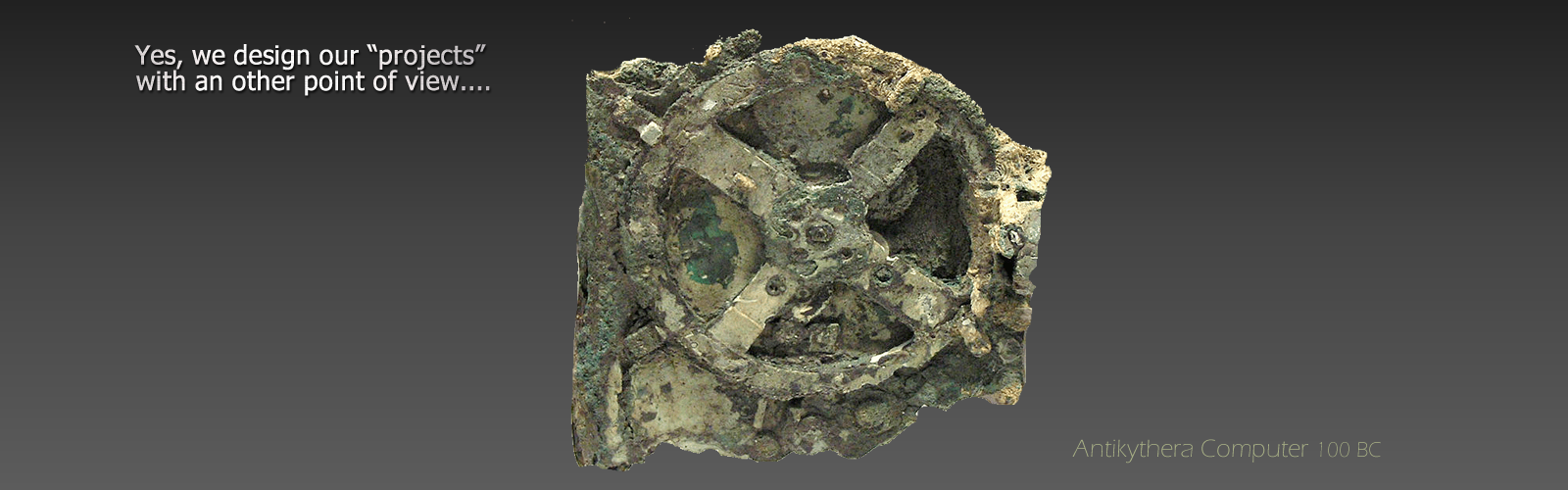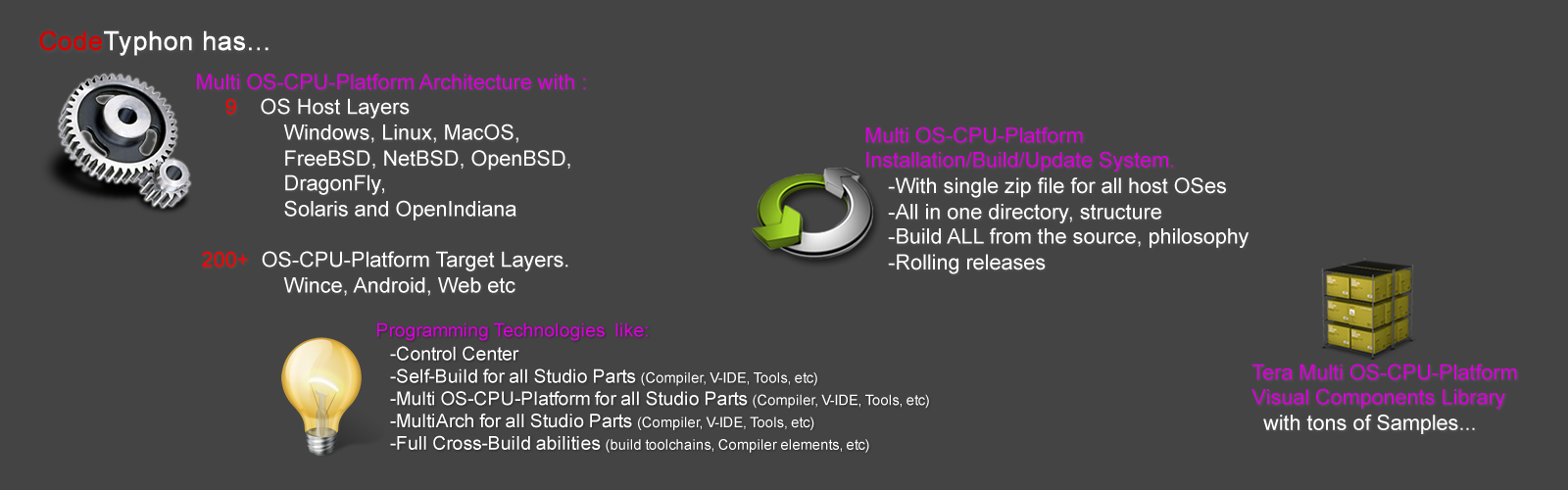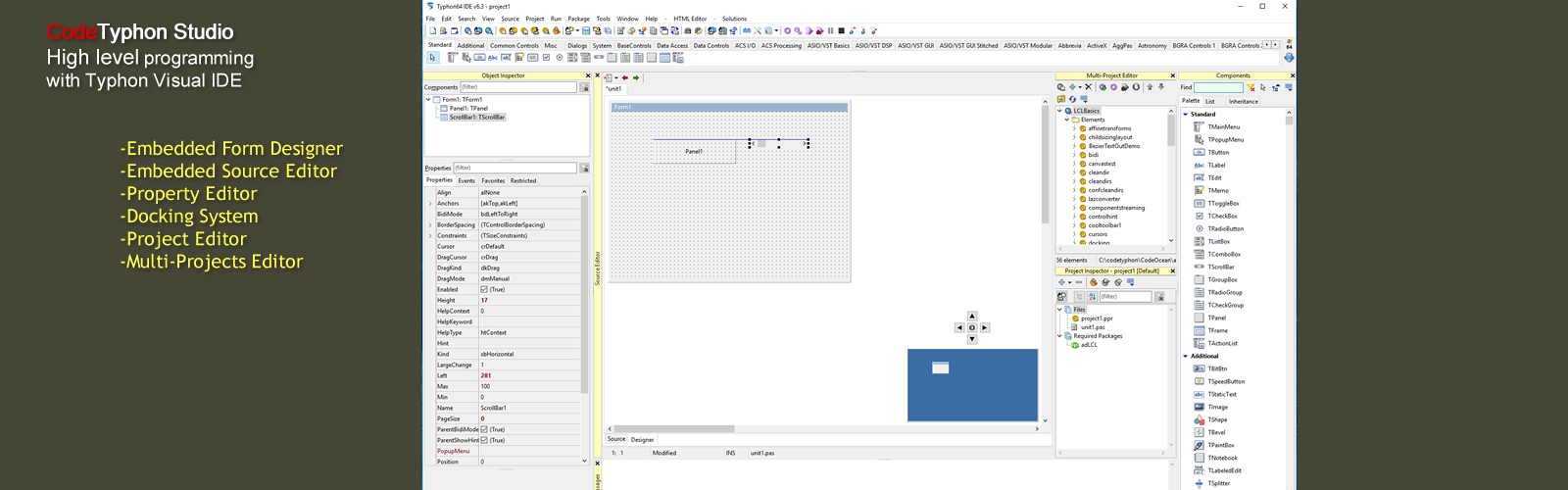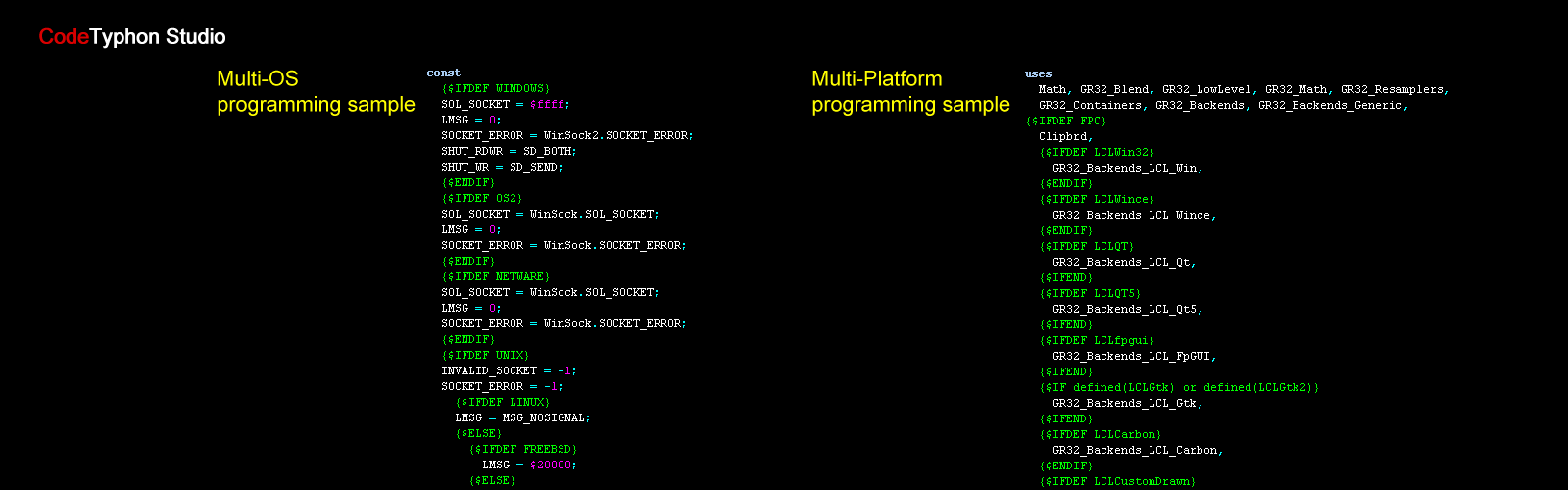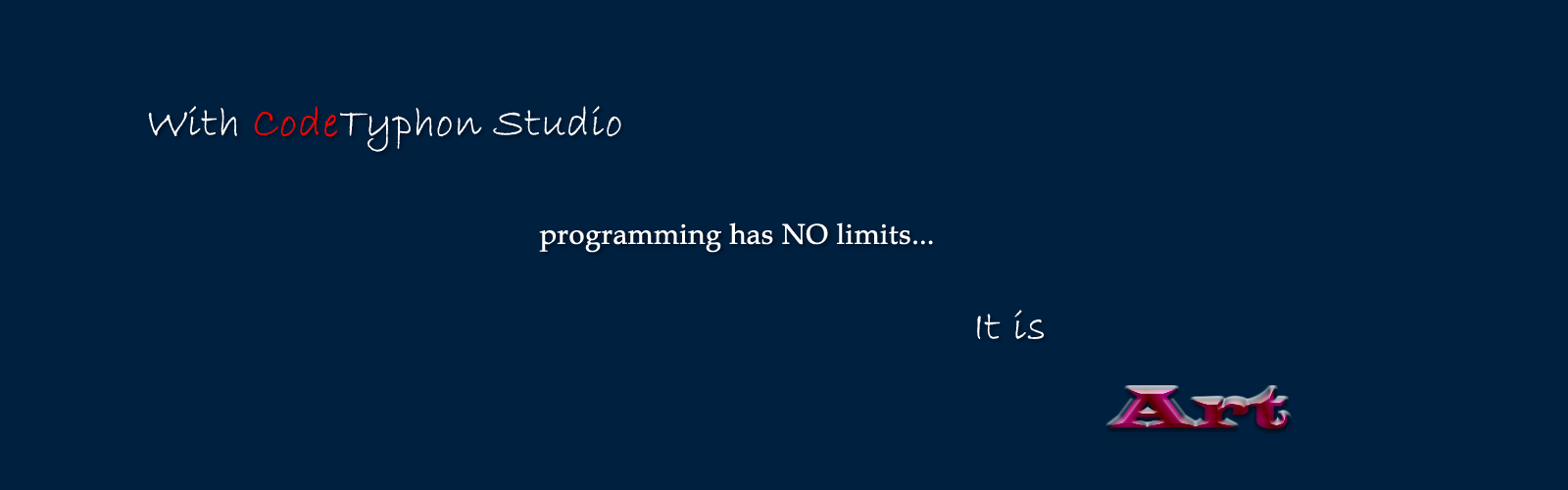## lz_PlotFunction

lz_PlotFunction

Library with simple Chart components.

Properties:

BackColor This is the background color of the plotting area
GridColor When you make this the same as Backcolor, the grid is invisible
LayerOption For easy plotting of a single layer this can be set to False. It is not possible to set this to True directly (see methods)
Margin The width of the border of the plot in pixels
PlotMode Three plotmodes are possible: pmBar, pmLine and pmDot
PlotPen Properties of the plotting pen. If LayerOption is False, then all sub-properties can be set. If LayerOption is True, then information about the plotting pen is transferred via the LayerOptions method (methods)
Title The Title is at the top center of the plot and uses the Font property
PlotBMP This is a bitmap holding the whole graph (see demo for its use)

The X-axis properties:

XLabel Label at the X-axis
XMarks When True, the X-axis has marks
XMarksFont Font used for the XMarks
XMarksInterval Interval between to lines in the grid (applies for linear scales only)
XMax Maximum value of X that is visible
XMin Minimal value of X that is visible
XScaleLog When TRUE the scale is logarithmic (the interval is determined by the component)

For the Y-axis the same applies.

Methods:

AddXY(X,Y:Extended) With this method you can add points (or lines) to the plot Example for i:= -10 to 10 do PlotPanel1.AddXY(i,i*i) This plots a parbola .The line is plotted with the PlotPen properties Note you can only plot a single layer.

AddXY(X,Y:Extended, Color:Tcolor,Layer:Integer) With this method it is possible to plot up to 8 lines in different colors, plotmodes and penwidths (see LayerOptions how to set linewidth and plotmodes)

LayerOptions(Layer:Integer;PlotMode:TPlotMode;PenWidth:Integer) Every Layer can use a different Plotmode and PenWidth When you call this method LayerOPtion will be set TRUE. When you want to use a single Layer again you have to set LayerOption False!

Autoscale(Layer:Integer) With this method you can adjust the size of the plot automaticly

ClearData Clears the data on the plot.

ConvertS2W(x,y: Extended; var WX,WY : Extended) Converts the screen-coordinates in X,Y to the real coordinates WX,WY. When X and Y are out of bounds, the function returns False, otherwise True

Freeze(Boolean) When Freeze(True) is executed all plotting is done on a invisible bitmap. After a Freeze(False) the whole plot is displayed. This produces flicker-free animations.

HideLayer(Layer:Integer) Use this to hide a single layer in the plot

UnHideLayer(Layer:Integer) Shows the hidden layer again N.B. When you add points to a hidden layer, the old layer is deleted and the new points are the only points in this layer!

Paint Repaints the whole Graph (is done automaticly by the system when necessairy)